# search

Search Modelithics component of interest

Since R2023a

## Syntax

``search(lobj,substrate)``
``search(lobj,substrate,Name=Value)``
``pobj = search(___)``

## Description

````search(lobj,substrate)` displays a window with two tabs. The first tab Search Results displays a list of all components in Modelithics SELECT+ Library™ components with a substrate `substrate`. The second tab Substrate lists all the substrates available in Modelithics SELECT+ Library. NoteThis function requires a Modelithics SELECT+ Library license. ```

example

````search(lobj,substrate,Name=Value)` specifies additional search criteria using name-value arguments. You can narrow down your component search by vendor name, type of passive component, and the value of the passive component.```

example

````pobj = search(___)` returns an array of `mdlxPart` object.```

## Examples

collapse all

Set up Modelithics SELECT+ Library™.

```mdlxSetup('C:\mdlx_library\SELECT') ```

Create a new Modelithics library object related to the library you set up.

```mdlx = mdlxLibrary; ```

Search the library for a component that you can mount on the `Rogers4350B4mil` substrate with the vendor name `ATC`.

```search(mdlx,'Rogers4350B4mil',Vendor='ATC') ```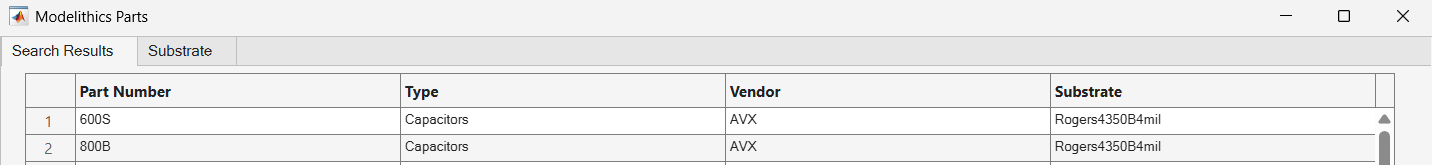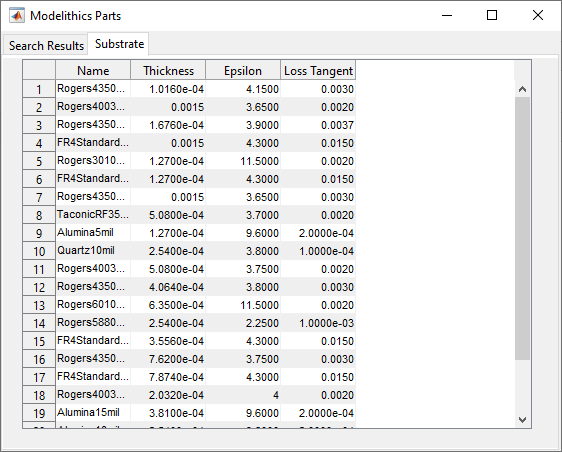The ATC 600S capacitor on a 4 mil Roger 4350B substrate fits the search criteria. Create a Modelithics component object that matches these characteristics.

```pobj = mdlxPart(mdlx,'600S','Capacitors','ATC','Rogers4350B4mil',Value=1.2e-12); ```

Create a circuit named `C_ATC_600S_Rogers4350B4mil`.

```hckt = circuit('C_ATC_600S_Rogers4350B4mil') ```Add the Modelithics component to the circuit.

```add(hckt,[1 2],pobj); ```

Set the ports of the circuit.

```setports(hckt, [1 0],[2 0]); ```

Display the Modelithics component.

```disp(pobj) ```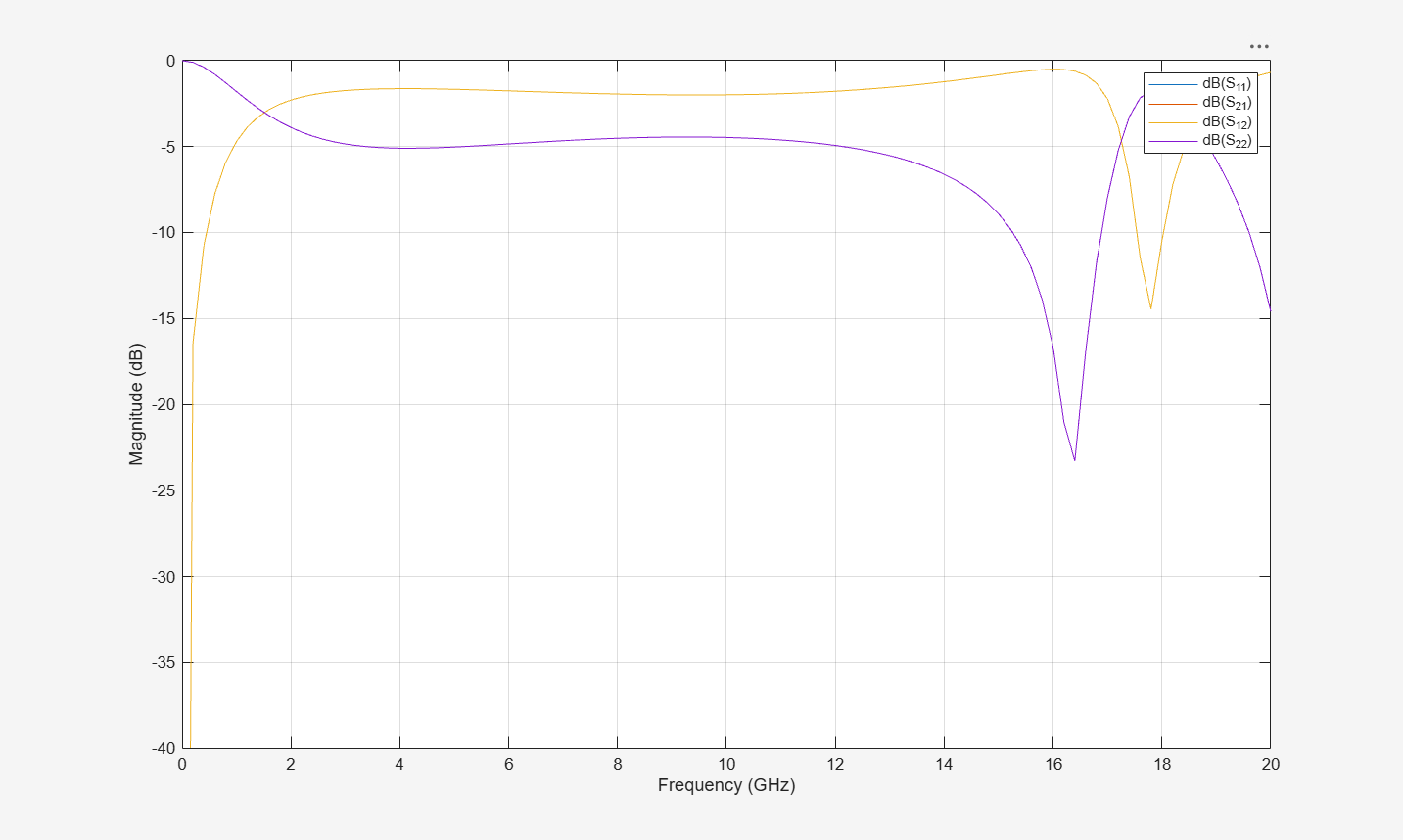Calculate the S-parameters of the circuit.

```S = sparameters(hckt,freq); ```

Plot the S-parameters characteristics.

```figure; rfplot(S) ```Set up Modelithics SELECT+ Library™ path.

```mdlxSetup('C:\mdlx_library\SELECT') ```

Create a new Modelithics library object with the Modelithics library set by the `mdlxSetup` object.

```mdlx = mdlxLibrary; ```

Search for capacitors mounted on an Alumina10mil substrate.

```search(mdlx,'Alumina10mil',Type='Capacitors') ```Narrow down your search by setting the capacitance to 10 pF and the vendor name to ATC.

```search(mdlx,'Alumina10mil',Type='Capacitors', Value=10e-12, Vendor='ATC') ```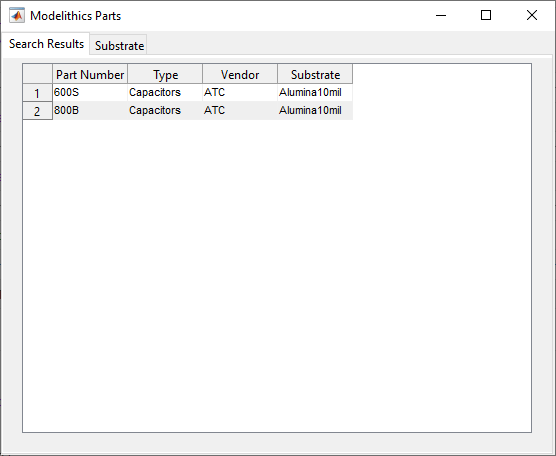Create an array of `mdlxPart` that matches the search criteria.

```pobj = search(mdlx,'Alumina10mil',Type='Capacitors', Value=10e-12, Vendor='ATC'); ```

Add the Modelithics component object to a circuit and compute and plot the S-parameters of the circuit.

```freq = linspace(0.05e9,20e9,500); for i = 1:length(pobj) ckt = circuit('example_circuit'); add(ckt,[1,2],pobj(i)); setports(ckt,[1 0],[2 0]); Sobj = sparameters(ckt,freq); figure; rfplot(Sobj) title([pobj(i).Vendor ' ' pobj(i).PartNumber ' ' ... pobj(i).Type ' = ' num2str(pobj(i).Value) ' on ' ... pobj(i).Substrate]) end ```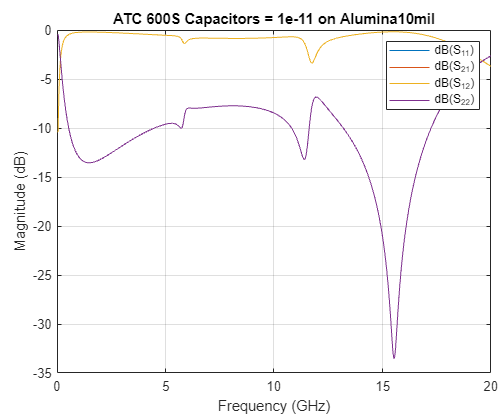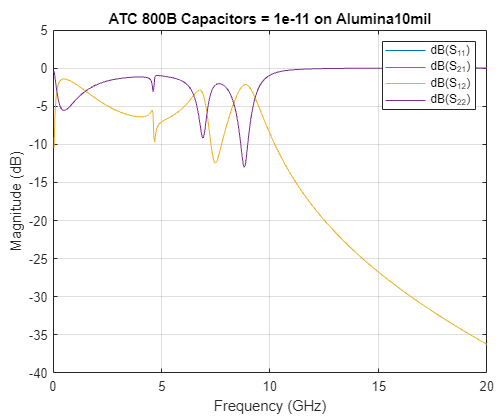## Input Arguments

collapse all

Modelithics® library object, specified as a `mdlxLibrary` object.

Name of the substrate in the Modelithics SELECT+ Library, specified as a string or character array.

### Name-Value Arguments

Specify optional pairs of arguments as `Name1=Value1,...,NameN=ValueN`, where `Name` is the argument name and `Value` is the corresponding value. Name-value arguments must appear after other arguments, but the order of the pairs does not matter.

Before R2021a, use commas to separate each name and value, and enclose `Name` in quotes.

Example: `search(mdlx,'Rogers4350B4mil',Vendor='ATC') `

Vendor name of the Modelithics component, specified either as a string or character vector.

Example: `Vendor='ATC'`

Type of passive components, specified as either `Capacitors`, `Inductors`, or `Resistors`.

Example: `Type='Resisitors'`

Value of the passive components, specified as a positive real scalar. You can specify the capacitance, inductance, and resistance values in farads, henries, or ohms, respectively.

Note

To search a component by its value, specify the `Value` argument with the `Type` argument.

Example: `Type='Capacitors',Value=1e-12`

## Output Arguments

collapse all

Modelithics component object, returned as an array of `mdlxPart` object.

## Version History

Introduced in R2023a